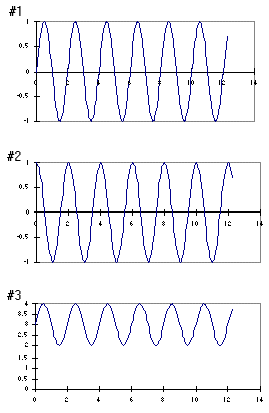Teaching Physics with the Physics Suite Edward F. Redish

Spring vs. string: graphs

Consider two physical systems:

• System A is a mass hanging from a light spring fixed at one end to a ringstand on a table above the floor;
• System B is a long spring of uniform density held under tension and able to move transversely in a horizontal plane.

In the figure at the left are shown three graphs labeled #1, #2, and #3, with unmarked axes. They could represent many quantities in the physical systems A and B.For the 5 physical quantities below, indicate which of the graphs could be obtained for the system indicated. If more than one graph applies, give all the possible choices. If none applies, write N. The height of the mass hanging from the spring (system A) above the ground as a function of time for some time interval after the mass has been set into oscillation. The transverse displacement from equilibrium of some portion of the long spring (system B) as a function of position at a particular instant of time while it is carrying a harmonic wave. The velocity of the mass hanging from the spring (system A) as a function of time given that its displacement from equilibrium as a function of time is given by graph #1 The transverse velocity of a small piece of the long spring (system B) as a function of time as a single pulse moves down the spring. The velocity of a small piece of the long spring (system B) as a function of time given that its displacement from equilibrium as a function of time is given by graph #2.

Not finding what you wanted? Check the Site Map for more information.